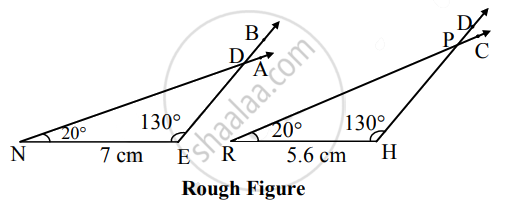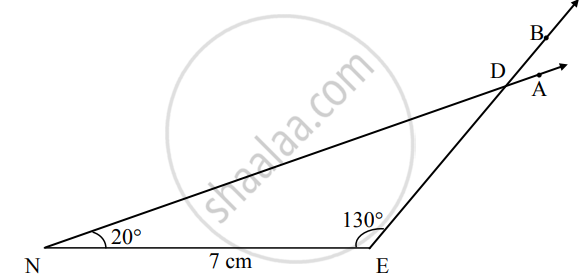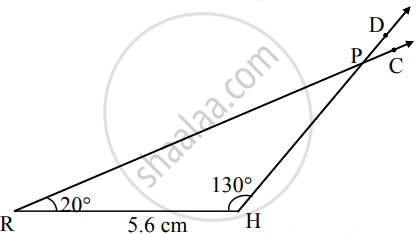# ΔRHP ~ ΔNED, In ΔNED, NE = 7 cm, ∠D = 30°, ∠N = 20° and HPED=45. Then construct ΔRHP and ΔNED - Geometry Mathematics 2

Chart
Diagram

ΔRHP ~ ΔNED, In ΔNED, NE = 7 cm, ∠D = 30°, ∠N = 20° and "HP"/"ED" = 4/5. Then construct ΔRHP and ΔNED

#### SolutionIn ∆NED, ∠D = 30°

and ∠N = 20°   ......(i) [Given]

∴ ∠E = 130°    .....(ii) [Remaining angle of a triangle]

∆RHP ∼ ∆NED

∴ "RH"/"NE" = "HP"/"ED" = "PR"/"DN"  .....[Corresponding sides of similar triangles]

∴ "RH"/7 = 4/5   ......[Given]

∴ RH = (4 xx 7)/5 = 5.6 cm

Also, ∠R = ∠N, ∠H = ∠E, ∠P = ∠D  ......(iii) [Corresponding angles of similar triangles]

∴ ∠R = 20°, ∠H = 130°, ∠P = 30°   ......[From (i),(ii) and (iii)]Steps of Construction:

 ∆NED ∆RHP i. Draw seg NE of 7 cm Draw seg RH of 5.6 cm ii. Draw a ray NA and EB such that ∠ANE = 20° and ∠BEN = 130°. Draw a ray RC and HD such that ∠CRH = 20° and ∠DHR = 130°. iii. Name the point of intersection of rays D. Name the point of intersection of rays P.
Is there an error in this question or solution?
Chapter 4: Geometric Constructions - Q.3 (B)

Share